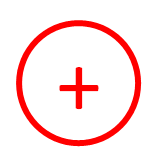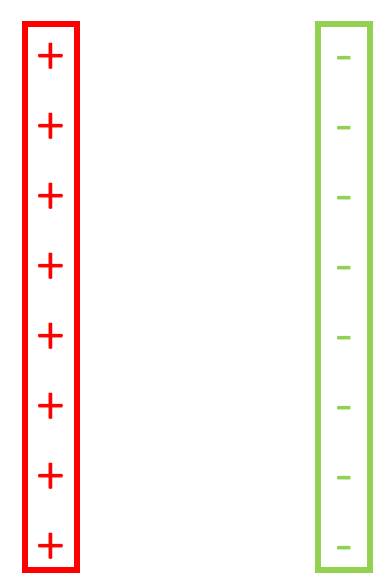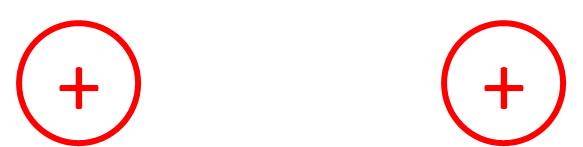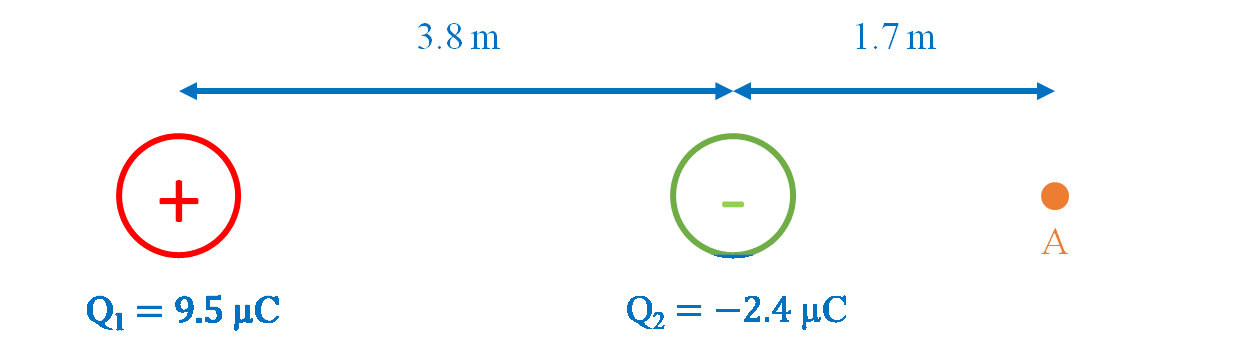# Electric field

### Electric field

#### Lessons

In this lesson, we will learn:
• Meaning of electric field and its relationship to electric force
• How to draw electric field line diagrams
• Solving problems with electric field
Notes:

• Electric field (E) is the electric force exerted by a charge Q on another charge q, per unit charge of q. It is a vector quantity.
• By convention, the direction of electric field vectors is defined as the direction that a positive test charge would move if placed in the field. Fe points in the same direction as E for positive charges, and in the opposite direction of E for negative charges.
• A test charge is a point charge with a very small magnitude. Test charges have a small magnitude charge so that the electric field of the test charge is negligible and does not affect the electric field that is being investigated.

Electric Field

$E= \frac{F_e}{Q}$
or equivalently, by substituting Coulomb's law:
$|E| = k \frac{|Q|}{r^2}$
$E:$ electric field, in newtons per coulomb (N/C)
$F_e:$ electric force, in newtons (N)
$q:$ charge that experiences the field, in coulombs (C)
$k:$ Coulomb's law constant, $9.00 \times 10^9 N\centerdot m^2 / C^2$
$|Q|:$ magnitude of charge that creates the field, in coulombs (C)
$r:$ distance from charge, in meters (m)

Coulomb's Law (Electric Force)

$|F_e| = k \frac{|Q_1 Q_2|}{r^2}$
$|F_e|:$ magnitude of electric force, in newtons (N)
$k:$ Coulomb's law constant, $9.00 \times 10^9 N\centerdot m^2 / C^2$
$|Q_1|, |Q_2|:$ magnitude of each charge, in coulombs (C)
$r:$ distance between charges, in meters (m)

• 1.
How to draw electric field line diagrams
Draw the electric field line diagrams for the following:
a)
Positive point chargeb)
Negative point chargec)
Parallel plates with equal magnitude chargesd)
Two point charges with opposite equal magnitude chargese)
Two point charges with positive equal magnitude point chargesf)
Two point charges with negative equal magnitude point charges• 2.
Calculations with electric field
A pair of positive and negative point charges are fixed in the following positions:a)
Find the electric field (magnitude and direction) at point A.

b)
A charge placed at A experiences a force of $5.9 \times 10^{-3} N$ [right]. Find the magnitude and polarity of the charge.